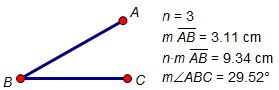﻿ Objects > Object Categories > Values

# Values

Navigation:  Objects > Object Categories >

# ValuesParameters, measurements, and calculations display numeric values and have many common characteristics.

The numeric values generated by measurements — and by calculations based on measurements — can be observed in order to discover relationships among objects in your sketch. In addition, the numeric values of all three kinds of objects can be used to define or control the behavior of your sketch in powerful and revealing ways.

Use numeric values to control transformed objects, plotted points, calculations, functions, and iterations.

## Units

The units of a value determine how it can be used in a sketch.

Distance values have units of centimeters, inches, or pixels, and can be used for translation.

Angle values have units of degrees or radians, and can be used for rotation or for the angle of a polar translation.

Dimensionless values have no units, and can be used as the scale factor for dilation.

Other values have units that don’t match these three categories (for instance, cm2 or pixels/radian).

## Using Values

All calculations, all parameters, and most measurements have a single numeric value. The values of any of these objects can be used in the same ways.

Change the value’s precision using the Value Properties panel. (To set the precision for new measurements, calculations, and parameters, choose )

Change the distance units or angle units of this value (and of all values in the sketch) using .

Change how the value is labeled using the Value Properties and Label Properties panels.

Include the value in a calculation or function by clicking the object while using the Calculator.

Choose Transform | Mark Distance to use a distance value as the marked distance for translation.

Choose Number | Tabulate to create a table which lists how one or more values change over time.

Choose Transform | Mark Angle to use an angle value as the marked angle for translation and rotation.

Choose Transform | Mark Scale Factor to use a value with no units as the marked scale factor for dilation.

Choose Graph | Define Unit Distance to use a distance value as the unit distance for a new coordinate system.

Select two values and choose Graph | Plot as (x, y) to plot a point with coordinates given by the selected values.

Use Transform | Iterate to Depth to define the depth of an iteration by a selected value.

Use Display | Color | Parametric Color to determine the color of an object.

Use Hot Text to include the value in a caption.